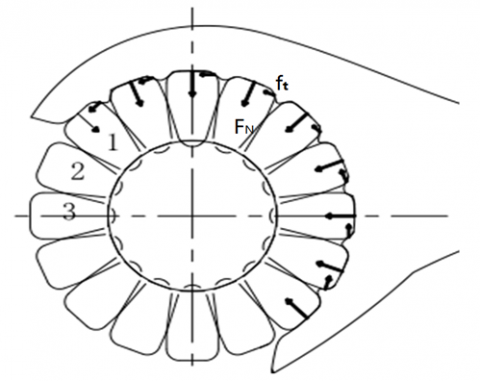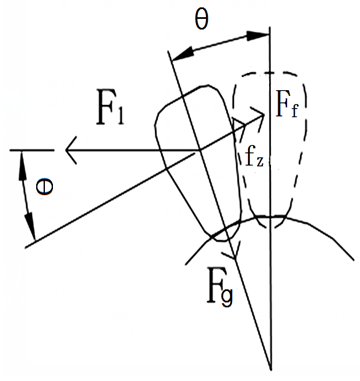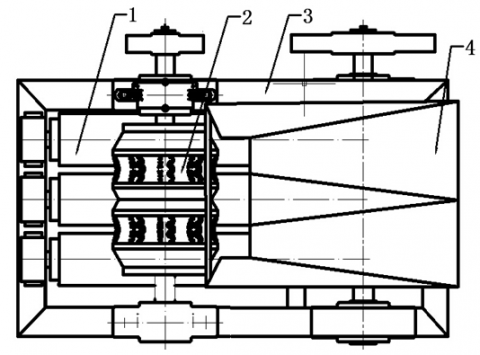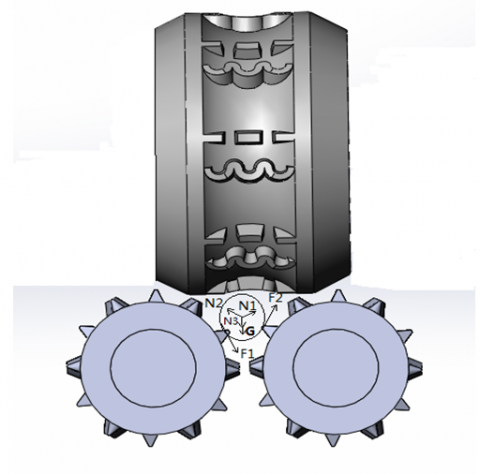# Bare Hands Threshing Stress Analysis and Bionics Bare Hand Threshing Device Test

Bare Hands Threshing Stress Analysis and Bionics Bare Hand Threshing Device Test

Ma Lei

College of Agricultural Engineering, Henan University of Science and Technology, Luoyang China,471003

Corresponding Author Email:
maleiemail@163.com
Page:
171-174
|
DOI:
https://doi.org/10.18280/mmep.030403
N/A
|
Accepted:
N/A
|
Published:
31 December 2016
| Citation

OPEN ACCESS

Abstract:

Bare hand threshing is the grain threshing method which was most original and most effective, minimal damage. This paper set up mechanics model of threshing with bare hands, analysis of stress grain, found that ordered threshing was the most reasonable way of threshing. Then design of bionic threshing device on the basis of bare hand threshing principle, and set up the model of bionic ear of corn threshing process and dynamic analysis. The analysis results show that the bionic threshing device can be in accordance with the bare hand threshing principle. Conduct water adaptability test of bare hand bionic threshing device, found that with the increase of moisture content, grain crushing rate increased gradually, and the net first decreases and then increases. Comprehensive consideration, 11.2% of water level for the best threshing condition, the crushing rate at the minimum, the threshing rate reached the maximum value.

Keywords:

bionics, seed corn, stress analysis, test, broken rate, removal rate

1. Introduction

At present in our country is still using the ordinary commercial corn seed threshing corn threshing machine, mainly depends on its high speed strike nail tooth corn ear and threshing, more than 700r/min in roller speed. The grain threshing damage, seriously affecting the seed germination rate and yield of maize. And it is not conducive to the development of precision agriculture, such as precision seeding requirements. Seed security is an important issue in relation to agricultural security. So reduce corn seed threshing damage become the main problems of mechanical threshing [1-8].

Bionics of bare hand threshing principle based on bare hands, with no injury to the embryo, the broken rate is low, the rate of removal is high, the adaptability of different varieties of corn and other advanced advantages, meet the target of seed corn thresher. [9-16]

2. Bare Hands Threshing Model Stress Analysis

The thick skin and subcutaneous tissues of the finger and palm are connected by fibrous septa to a network containing less fat groups. When the finger touches the corn, the palm and fingers enter into the gap among the grains. This process was equivalent to the effect of flexible materials on the corn grain surface and considerably reduces the friction and wear on the maize grain, thereby protecting the skin. At the same time, the finger palm surface distribution of a large number of fingerprints, can produce enough friction Thus, the gap between the fingers and the grain meat produce a thrust force of (n-1) ft, and The friction force of the finger to the grain was nμFy, and the number of grains in contact with the palm was represented by n, in figure 1.

## 1.pngFigure 1.Schematic diagram of bare hand threshing

Assuming that the friction among grain seeds was fz, the connection force between grains and the ear was Fg, and the angle between the two grains was θ. At this point, the finger only has access to the final grain 1, which was then used for the study of dynamic equilibrium equation.

$\sum{{{\text{F}}_{\text{z}}}\text{=}{{\text{F}}_{\text{1}}}\text{+}{{\text{f}}_{\text{z}}}\text{+}{{\text{F}}_{\text{f}}}\text{-}{{\text{f}}_{\text{t}}}\text{- }\!\!\mu\!\!\text{ }{{\text{F}}_{\text{n}}}\text{=0}}$                         (1)

Therefore

${{\text{F}}_{\text{1}}}\text{=}{{\text{f}}_{\text{t}}}\text{+ }\!\!\mu\!\!\text{ }{{\text{F}}_{\text{n}}}\text{-}{{\text{f}}_{\text{z}}}\text{-}{{\text{F}}_{\text{f}}}$                                               (2)

Taking grain 2 as the research object, the dynamic equilibrium equation was studied by the following figure 2:

## 2.pngFigure 2. Grain 2 stress diagram

$\sum{\text{F}=}{{\text{F}}_{\text{2}}}\text{-}{{\text{F}}_{\text{n}}}\text{cos }\!\!\theta\!\!\text{ }+{{\text{f}}_{\text{z}}}+{{\text{F}}_{\text{f}}}$                (3)

The stress of the grain 2 was:

\begin{align} & {{F}_{\text{2}}}={{\text{f}}_{\text{t}}}\text{cos}\theta +\mu \text{cos}\theta {{F}_{\text{n}}}-1+\text{cos}\theta {{\text{f}}_{\text{z}}} \\ & \text{-}1+\text{cos}\theta {{F}_{\text{f}}} \\ \end{align}                           (4)

In the same way, the stress of grain 3 was:

\begin{align} & {{\text{F}}_{\text{3}}}\text{=}{{\text{f}}_{\text{t}}}\text{co}{{\text{s}}^{\text{2}}}\text{ }\!\!\theta\!\!\text{ + }\!\!\mu\!\!\text{ }{{\text{F}}_{\text{n}}}\text{co}{{\text{s}}^{\text{2}}}\text{ }\!\!\theta\!\!\text{ -} \\ & \text{(1+cos }\!\!\theta\!\!\text{ +co}{{\text{s}}^{\text{2}}}\text{ }\!\!\theta\!\!\text{ )}{{\text{f}}_{\text{z}}}\text{-(1+cos }\!\!\theta\!\!\text{ +co}{{\text{s}}^{\text{2}}}\text{ }\!\!\theta\!\!\text{ )}{{\text{F}}_{\text{f}}} \\ \end{align}                       (5)

The connection force between the grain and the corn cob was Fg, and the friction coefficient of the grain in the y-axis direction was μ. The force applied to grain 1 was pressure Fy and thrust ft, and the sum of force was F.

Therefore,

$\text{ F = }\!\!\mu\!\!\text{ }{{\text{F}}_{\text{y}}}\text{ + }{{\text{f}}_{\text{t}}}$                 (6)

When using grain 1 for the study, the kinetic equilibrium equation was given by:

$\sum{F=F_{\text{t}}^{1}}\text{-}\text{ }\!\!\mu\!\!\text{ }{{\text{F}}_{\text{1}}}\text{-}{{F}_{\text{g}}}=0$                 (7)

Then the stress of the grain 1 was:

$F_{\text{t}}^{1}=\text{ }\!\!\mu\!\!\text{ F- }\!\!\mu\!\!\text{ }{{\text{f}}_{z}}\text{- }\!\!\mu\!\!\text{ }{{\text{F}}_{f}}\text{-}{{\text{F}}_{g}}$                     (8)

Taking grain 2 as the research object, the dynamic equilibrium equation was:

$\sum{F=F_{\text{t}}^{2}}\text{-}\text{ }\!\!\mu\!\!\text{ }{{\text{F}}_{\text{2}}}\text{-}{{F}_{g}}=0$                                          (9)

Then the stress of the grain 2 was:

\begin{align} & \text{F}_{\text{t}}^{\text{2}}\text{= }\!\!\mu\!\!\text{ Fcos }\!\!\theta\!\!\text{ - }\!\!\mu\!\!\text{ (1+cos}\theta {{\text{f}}_{z}} \\ & \text{ - }\!\!\mu\!\!\text{ (1}+\text{cos }\!\!\theta\!\!\text{ }{{\text{F}}_{z}}\text{-}{{\text{F}}_{g}} \\ \end{align}                           (10)

In the same way, the stress of grain 3 was:

\begin{align} & F_{\text{t}}^{3}=\mu F\text{co}{{\text{s}}^{2}}\theta \text{-}\mu (1+\text{cos}\theta +\text{co}{{\text{s}}^{2}}\theta ){{f}_{z}} \\ & -\mu (1+\text{cos}\theta +\text{co}{{\text{s}}^{2}}\theta ){{F}_{z}}\text{-}{{F}_{g}} \\ \end{align}            (11)

Grain threshing condition was:

${{\text{F}}_{t}}\text{= }\!\!\mu\!\!\text{ F-}{{\text{F}}_{g}}\text{0}$                    (12)

Threshing condition of grain 1 was:

$\text{F}{{\text{f}}_{\text{z}}}\text{+}{{\text{F}}_{z}}\text{+}{{\text{F}}_{g}}\text{/ }\!\!\mu\!\!\text{ }$                         (13)

Threshing condition of grain 2 was:

\begin{align} & F>1+\cos \theta {{f}_{\text{z}}}/\text{cos}\theta + \\ & 1+\cos \theta {{F}_{\text{f}}}/\text{cos}\theta +{{F}_{\text{g}}}/\mu \text{cos}\theta \\ \end{align}                           (14)

Threshing condition of grain 3 was:

\begin{align} & \text{F}\text{ }\!\![\!\!\text{ (1+cos }\!\!\theta\!\!\text{ +co}{{\text{s}}^{\text{2}}}\text{ }\!\!\theta\!\!\text{ }{{\text{f}}_{\text{z}}}\text{/cos }\!\!\theta\!\!\text{ +} \\ & \text{(1+cos }\!\!\theta\!\!\text{ +co}{{\text{s}}^{\text{2}}}\text{ }\!\!\theta\!\!\text{ }{{\text{F}}_{\text{f}}}\text{/cos }\!\!\theta\!\!\text{ +}{{\text{F}}_{g}}\text{ }\!\!]\!\!\text{ /co}{{\text{s}}^{\text{2}}}\text{ }\!\!\theta\!\!\text{ } \\ \end{align}                                 (15)

From this equation, cosθ > 0, which indicates that the threshing force for grain 3 was higher than the force required for threshing grain 2. Moreover, the force required for threshing grain 2 was higher than the required threshing grain1. Threshing of grain 1 requires minimum force, thus grain 1 was the ideal state when threshing. As such, force should be first applied to separate grain 1 and the ear before acting on grains 2 and 3. Grains were threshed down in each row, and the process was continued to the next row.

3. The Working Principle of Bionic Threshing Device

Design of bionic threshing device on the basis of bare hand threshing principle, shown in figure 3. Corn ear random feed inlet, the axis of the ear and the differential speed roller was not parallel, adjusted in the feed inlet, and correct their position along the axis parallel to the two differential roller axis movement step by step. The corn ear was pushed in the imitated bare hand threshing space, resulting in complete bionic of threshing with bare hands in the threshing and differential speed rollers; hence, differential threshing induces less damage to the corn ear. Grains fell off in the gap among the differential rollers and recovered by the recovery port grain. Differential roller speed was positioned along the outer axial discharge of the corn cob machine. This machine threshes the corn ear by force using differential speed rollers along the axial order without breaking the corn cob, thereby saving cost due to the cleaning system and reducing power consumption.

## 3.pngFigure 3. Test bench structure: 1.Differential speed roll 2. Threshing roller 3. Frame 4. Feed inlet

4. Dynamic Analysis on Threshing Device of the Bionic Ear Threshing Model

The corn ear was placed between the roller and the bottom two threshing rollers. The results of corn ear stress analysis are shown in figure 4.

## 4.pngFigure 4. Corn ear positive force analysis chart

N1 and N2 represent the support force on the corn ear caused by the differential speed rollers. The clockwise rotation of the bionic unit on these rollers applied force F1 and F2, drove the counterclockwise rotation of corn ear, and threshed the grain rows and rows under the gravity and the threshing roller pressure. As shown in Figure 5, the threshing roller threshing unit of imitation bare hands rotating in a counter clockwise direction would produce spatial resultant force F on the rotating corn ear. Radial component of the spatial resultant force F would be threshed by row, in accordance with the radial. And axial component of the spatial resultant force F would be threshed by ring, in accordance with the radial, from the front to the back. Friction f applied in corn ear horizontal backward drive backward movement of corn ear, in order to ensured the threshing of not threshing part under the threshing roller.

## 5.pngFigure 5. Corn ear lateral force analysis chart

5. Bionics Bare Hand Threshing Device Test

Conduct water adaptability test of bare hand bionic threshing device, test for maize varieties Zhengdan 958, differential roller speed of 100r/min, threshing roller speed of 250 r/min, selected water content were 11.2%, 14.5%, 17.5% and 19.8% of the four representative water level test, the results are shown in the following table 1.

As shows of table 1, with the increase of moisture content, grain crushing rate increased gradually, and the net first decreases and then increases. Comprehensive consideration, 11.2% of water level for the best threshing condition, the crushing rate at the minimum, the threshing rate reached the maximum value.

Table1. Threshing device moisture test table

 Moisture content Broken rate Removal rate 11.2 0.368 99.2 14.5 0.750 97.5 17.5 0.835 82.1 19.8 2.94 94.9
6. Conclusions

(1) Stress analysis of grain threshing process by bare hands, one by one in order to grain threshing corn is the ideal state. At this time, the required force was the smallest, and the force between grains was the least, the efficiency was the highest.

(2) Design a threshing device based on bare hands and stress analysis with the threshing model, found that the device can achieve the purpose of ordered threshing, achieve the bare hand threshing effect.

(3) Conduct water adaptability test of bare hand bionic threshing device, discovered that 11.2% of water level for the best threshing condition, the crushing rate at the minimum, the threshing rate reached the maximum value.

References

 S. Petkevichius, L. Shpokas and H. D. Kutzbach, “Investigation of the maize ear threshing process,” Biosystems Engineer, 99, no. 4, pp. 532- 539, 2008.

 E. A. Ajav and Adejumo B. A., “Performance evaluation of an Okra Thresher,” The CIGR Ejournal.Manuscript, PM 04 006 Vol.VII., October 2005.

 M. Bulent Coskun, et.al., “Physical properties of sweet corn seed (Zea mays saccharata Sturt),” Journal Of Food Engineering, vol. 74, no. 06, pp. 523-528, 2006.

 Tastra, I.K., Ginting, Erliana and Merx Richard, “Determination of the optimum moisture content for shelling maize using local shellers,” AMA, Agricultural Mechanization in Asia, Africa and Latin America, vol.  37, no. 2, pp. 51-57, 2006.

 Volkovas, Vitalijus, Petkevicius, Sigitas and Špokas, Liudas, “Establishment of maize grain elasticity on the basis of impact load,” Mechanika, vol. 62, no. 6, pp. 64-67, 2006.

 S. O. Nkakini, M. J. Ayotamuno, G.P.D. Maeba, S.O.T. Ogaji and S. D., “Probert. Manually-powered continuous-flow maize-sheller,” Applied Energy, no. 84, pp. 1175-1186, 2007.

 S. Petkevichius, L. Shpokas and H.-D. Kutzbach, “ Investigation of the maize ear threshing process,” Biosystem Engineering, no. 99, pp. 532–539, 2008.

 Tastra, I. K., “ Designing and testing an improved maize sheller,” AMA, Agricultural Mechanization in Asia, Africa and Latin America, vol. 40, no. 1, pp. 12-17, 2009.

 Singh, S P., Singh, Surendra and Singh, Pratap, “Ergonomics in developing hand operated maize Dehusker-Sheller for farm women,” Applied Ergonomics, 2011.

 Yang Yougang, and Liu Yingchun, “Design of a bionic ditch digger,” Transactions of the Chinese Society for Agricultural Machinery, vol. 35, no. 1, pp. 65-68, 2004.

 Li Xinping, Li Yuzhu, Gao Hang, et al., “Bionic threshing process analysis of seed corn kernel,” Transactions of the Chinese Society for Agricultural Machinery, vol. 42, no. 2, pp. 99-103, 2011.

 Li Xinping, Ma Yidong, Jin Xin and Gao Lianxing, “Design and test of seed corn bionic thresher,” Transactions of the Chinese Society for Agricultural Machinery, vol. 46, no. 7, pp. 97-101, 2015.

 Li Xinping, Ma Fuli and Gao Lianxing, “The design of differential type corn seed threshing machine,” Ransactions ofthe Chinese Society for Agricultural Machinery, vol. 9, no. 8, pp. 192-195, 2008.

 Li Xinping, Liu Ying, Ma Yidong, et al., “Effect of gap direction among corn ear kernels on the circulating dint decline,” Journal of Agricultural Mechanization Research, vol. 37, no. 1, pp. 183-187, 2015.

 Li Xinping, Du Zhe, Ma Yidong, et al., “Bare hand threshing experiment on corn ear kernel,” International Agricultural Engineering Journal, vol. 23, no. 3, pp. 74-80, 2014.

 Zhao Wuyun and Wang Guangwan, “Research and design on low damage corn seed sheller,” Machine research and Application, vol. 23, no. 1, pp. 132-134, 2010.

 Liu Jian’an and Li Weijie, “The designof spiral corn sheller by extruding and rubbing method,” Journal of Agricultural Mechanization Research, vol. 32, no. 8, pp. 82-84, 2010.

 Wang Jing, Nie Ying and Gong Yuanjuan, “Experimention on thresher of maize pair seeds,” Transactions of the Chinese Society for Agricultural Machinery, vol. 42, no. 2, pp. 104-108, 2011.

 He Shuguo, Che Gang and Wan Lin, “Manufacture and study on 5TY-10A type corn seed thresher,” Journal of Heilongjiang August First Land Reclamation University, vol. 18, no. 3, pp. 55-59, 2006.

 Li Y unyan and Hu Chuanrong, Experiment Dasign and Date Processing, Beijing: Chemical Industry Press, 2004.

 Folarin Alonge, AKosemani and Babajide S., “ Development of a guinea corn thresher,” American Society of Agricultural and Biological Engineers Annual International Meeting, no. 2, pp. 1281-1288, 2011.

 Tastra, I.K., “Designing and testing an improved maize sheller,” AMA, Agricultural Mechanization in Asia, Africa and Latin America, vol. 40, no. 1, pp. 12, 2009.

 Folarin Alonge, A. Kosemani and Babajide S., “ Development of a guinea corn thresher,” American Society of Agricultural and Biological Engineers Annual International Meeting, no. 2, pp. 1281-1288, 2011.

 Fandohan P., et al., “Impact of mechanical shelling and dehulling oil fusarium infection and fumonisin contamination in maize,” Food Additives and Contaminants, vol. 23, no. 4, pp. 415-421, 2006.